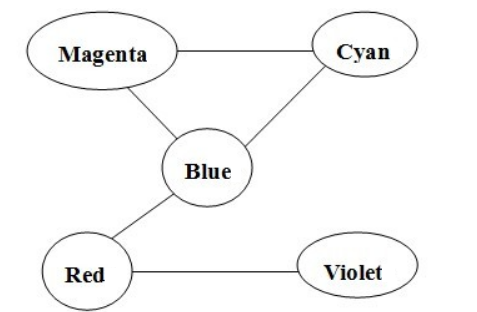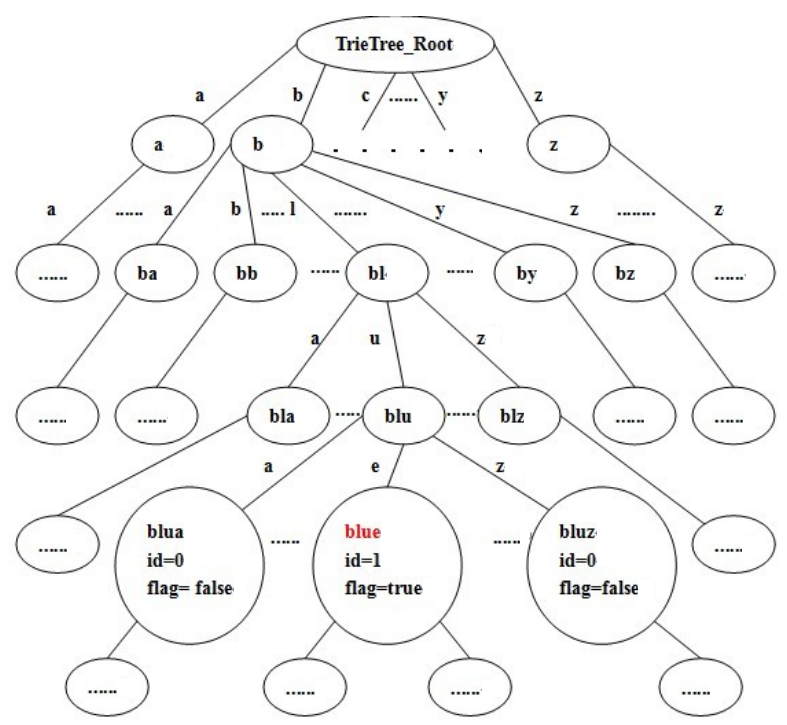# POJ 2513 - Colored Sticks

## 解题思路

• ① 图是连通的；
• ② 所有节点的度为偶数，或者有且只有两个度为奇数的节点。

``````Blue=3
Red=2
Violet=1
Cyan=2
Magenta=2``````• 字典树
• 欧拉路径：其中又考察了判断是否为连通图
• 并查集 及其优化方法（路径压缩）

• POSSIBLE： 奇度数节点个数 `==0``==2` 且 图连通
• IMPOSSIBLE：奇度数节点个数 `==1``>=3` 或 图不连通

## AC 源码

``````/* TrieTree + MergeSet + EulerPath*/

//Memory Time
//77460K 2047MS

#include<iostream>
using namespace std;

const int large=500000;  //25W条棒子，有50W个端点

class TrieTree_Node   //字典树节点
{
public:
bool flag;   //标记到字典树从根到当前节点所构成的字符串是否为一个(颜色)单词
int id;     //当前颜色(节点)的编号
TrieTree_Node* next;

TrieTree_Node()   //initial
{
flag=false;
id=0;
memset(next,0,sizeof(next));  //0 <-> NULL
}
}root;   //字典树根节点

int color=0;  //颜色编号指针，最终为颜色总个数

int degree[large+1]={0};   //第id个节点的总度数
int ancestor[large+1];   //第id个节点祖先

/*寻找x节点的最终祖先*/

int find(int x)
{
if(ancestor[x]!=x)
ancestor[x]=find(ancestor[x]);   //路径压缩
return ancestor[x];
}

/*合并a、b两个集合*/

void union_set(int a,int b)
{
int pa=find(a);
int pb=find(b);
ancestor[pb]=pa;   //使a的祖先 作为 b的祖先
return;
}

//利用字典树构造字符串s到编号int的映射

int hash(char *s)
{
TrieTree_Node* p=&root;  //从TrieTree的根节点出发搜索单词(单词不存在则创建)

int len=0;
while(s[len]!='\0')
{
int index=s[len++]-'a';  //把小写字母a~z映射到数字的1~26，作为字典树的每一层的索引

if(!p->next[index])  //当索引不存在时，构建索引
p->next[index]=new TrieTree_Node;

p=p->next[index];
}

if(p->flag)  //颜色单词已存在
return p->id;  //返回其编号
else   //否则创建单词
{
p->flag=true;
p->id=++color;
return p->id;   //返回分配给新颜色的编号
}
}

int main(void)
{
/*Initial the Merge-Set*/

for(int k=1;k<=large;k++)   //初始化，每个节点作为一个独立集合
ancestor[k]=k;  //对于只有一个节点x的集合，x的祖先就是它本身

/*Input*/

char a,b;
while(cin>>a>>b)
{
/*Creat the TrieTree*/

int i=hash(a);
int j=hash(b);  //得到a、b颜色的编号

/*Get all nodes' degree*/

degree[i]++;
degree[j]++;   //记录a、b颜色出现的次数(总度数)

/*Creat the Merge-Set*/

union_set(i,j);
}

/*Judge the Euler-Path*/

int s=find(1);  //若图为连通图，则s为所有节点的祖先
//若图为非连通图，s为所有祖先中的其中一个祖先

int num=0;  //度数为奇数的节点个数

for(int i=1;i<=color;i++)
{
if(degree[i]%2==1)
num++;

if(num>2)   //度数为奇数的节点数大于3，欧拉路必不存在
{
cout<<"Impossible"<<endl;
return 0;
}

if(find(i)!=s)   //存在多个祖先，图为森林，不连通
{
cout<<"Impossible"<<endl;
return 0;
}
}

if(num==1) //度数为奇数的节点数等于1，欧拉路必不存在
cout<<"Impossible"<<endl;
else       //度数为奇数的节点数恰好等于2或不存在，存在欧拉路
cout<<"Possible"<<endl;

return 0;
}``````

## 相关资料

上一篇POJ 3982 - The Fibonacci sequence
POJ 3982 - The Fibonacci sequence Time: 1000MS Memory: 65536K 难度: 初级 分类: 高精度算法 问题描述变种的大数斐波那契数列 解题思路水题，直接加就可以了，循环使用4个大数
2011-04-21POJ 3096 - Surprising Strings
POJ 3096 - Surprising Strings Time: 1000MS Memory: 65536K 难度: 中级 分类: 基础算法 问题描述定义D-pairs表示取字符串s中相距为D的两个字母所构成的字母对，该字母对中两
2011-04-15
目录